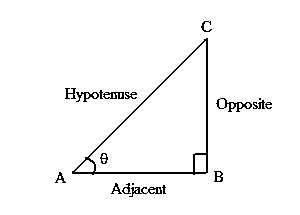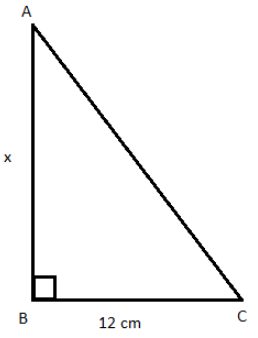# Sine Formula

To calculate the angle of the right-angled triangle, sine formula is used. The relation between the sides and angles of the right angle is shown through this formula. Sine is the ratio of the opposite side to the hypotenuse side of the right triangle. The longest side is the hypotenuse and the opposite side of the hypotenuse is the opposite side. In trigonometry, Sin is the shorthand of sine function.The Sine Angle Formula is,

$\large Sin\,\theta=\frac{Opposite}{Hypotenuse}$

### Solved Examples

Question 1: Calculate the sine angle of a right triangle whose opposite side and hypotenuse are 10 cm and 12 cm respectively?

Solution:

Given, Opposite side = 10 cm Hypotenuse = 12 cm

Using the formula:

$$\begin{array}{l}sin \theta\end{array}$$

=
$$\begin{array}{l}\frac{Opposite}{Hypotenuse}\end{array}$$

$$\begin{array}{l}sin \theta\end{array}$$

=
$$\begin{array}{l}\frac{10 cm}{12 cm}\end{array}$$

$$\begin{array}{l}sin \theta\end{array}$$

= 0.83

Question 2: If sin A = 0.5, then find the value of x from the following figure.Solution:
Given,
Sin A = 0.5 = 5/10 = ½
We know that sin θ = Opposite/Hypotenuse
BC/AC = ½
12/AC = ½
AC = 12 × 2 = 24 cm
By Pythagoras theorem,

AC2 = AB2 + BC2
242 = x2 + 122
x2 = 576 – 144
x2 = 432
x = √452 cm

x=20.78 cm